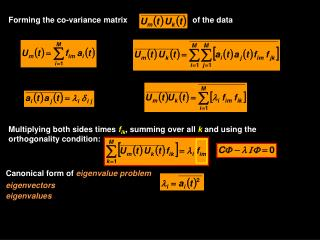Download PresentationForming the co-variance matrix

# Forming the co-variance matrix

Download Presentation## Forming the co-variance matrix

- - - - - - - - - - - - - - - - - - - - - - - - - - - E N D - - - - - - - - - - - - - - - - - - - - - - - - - - -
##### Presentation Transcript

1. Forming the co-variance matrix of the data Multiplying both sides times fik, summing over all k and using the orthogonality condition: Canonical form of eigenvalue problem eigenvectors eigenvalues

2. I is the unit matrix and  are the EOFs Eigenvalue problemcorresponding to a linear system:

3. Matrix = [6637,18] rows > columns

4. Matrix ul = [6637,18] >> uc=cov(ul); >> u1=ul(:,1); >> sum((u1-mean(u1)).^2)/(length(u1)-1) ans = 9.6143 >> u2=ul(:,2); >> sum((u1-mean(u1)).*(u2-mean(u2)))/(length(u1)-1) ans = 10.1154

5. Covariance Matrix Maximum covariance at surface

6. >> uc=cov(ul); >> [v,d]=eig(uc); eigenvalues (or lambda) >> lambda=diag(d)/sum(diag(d));

7. >> uc=cov(ul); >> [v,d]=eig(uc);

8. >> uc=cov(ul); >> [v,d]=eig(uc); >> v=fliplr(v);

9. Mode 2 13.2% Mode 1 85.3%

10. >> ts=ul*v; ts=[6637,18] Mode 1 85.3% Mode 2 13.2% Mode 2 13.2% Mode 1 85.3%

11. >>for k=1:nz vt(k,:,:)=ts(:,k)*v(:,k)'; end vt=[18, 6637,18] mode # evolution in time time series # >> v1=squeeze(vt(1,:,:))’; >> v2=squeeze(vt(2,:,:))’; Depth (m)

12. Depth (m)

13. Depth (m)

14. Complex Empirical Orthogonal Functions – James River Data u v Linear combination of spatial predictors or modes that are normal or orthogonal to each other

15. Rotated 49 degrees Streamwise Cross-stream

16. Mode 1 96.5% Mode 1

17. Mode 2 2.5% Mode 2

18. Streamwise Mode 1 96.5% cross-stream

19. Mode 1 96.5% Principal-axis Mode scaling cross-axis

20. Mode 2 2.5% Streamwise Mode scaling Cross-stream

21. Mode 2 2.5% Streamwise Mode scaling cross-stream

22. Mode 1 75% m/s m/s m/s

23. Mode 2 22% m/s m/s m/s

24. Phase of EOFS Mode 2 Depth (m) Mode 1 radians

25. streamwise cross-stream

26. streamwise cross-stream